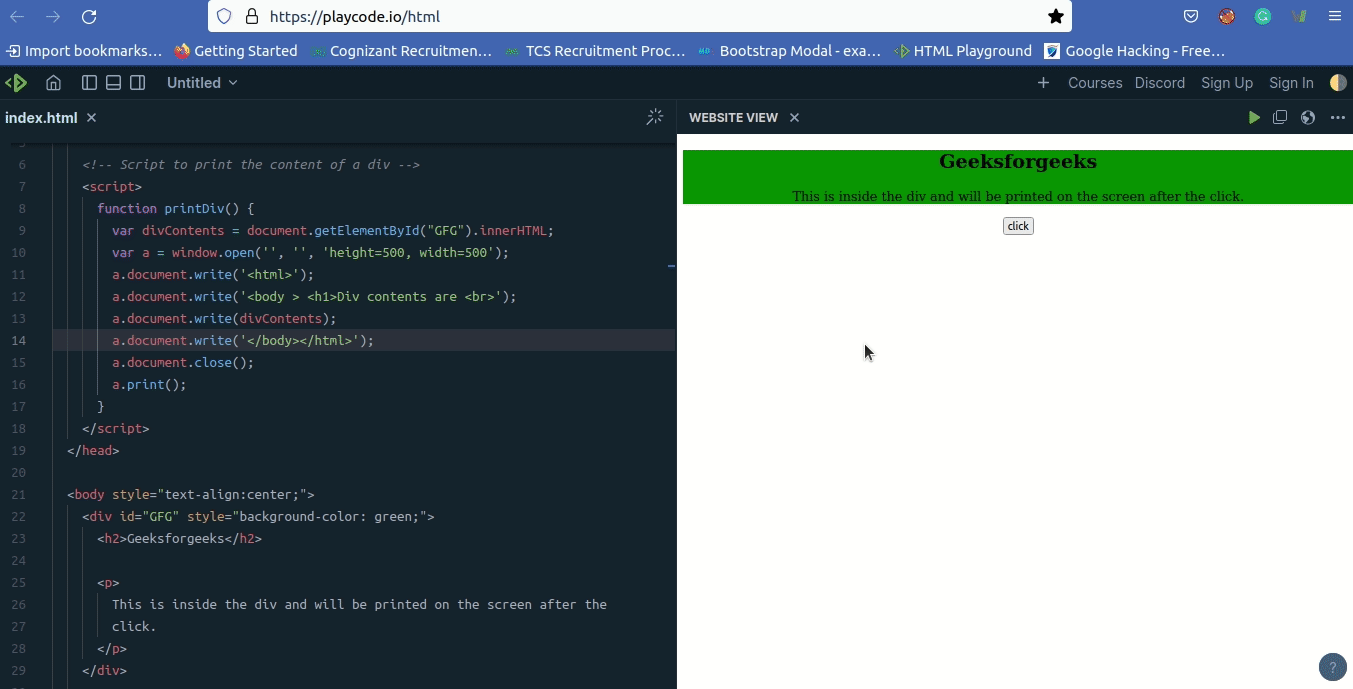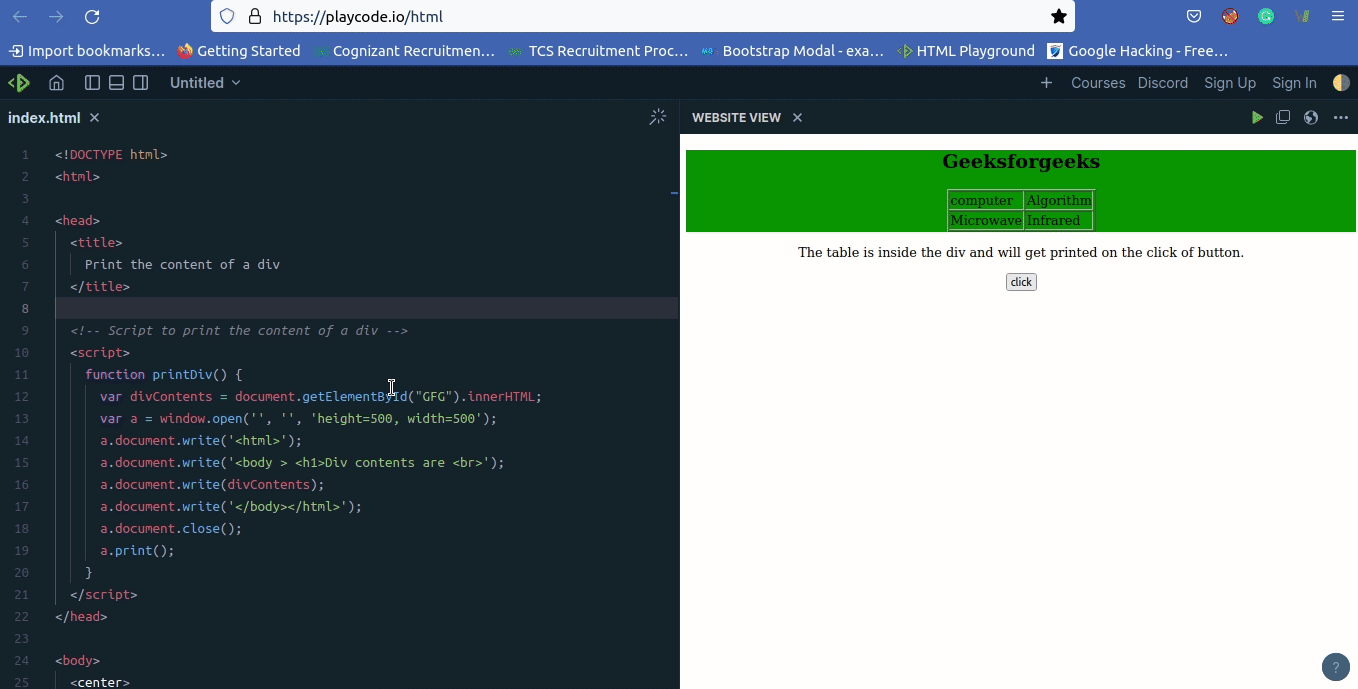Open In App

# Print the content of a div element using JavaScript

To print the content of div in JavaScript, first store the content of div in a JavaScript variable and then the print button is clicked. The contents of the HTML div element to be extracted. Then a JavaScript popup window is created and the extracted contents of the HTML div elements are written to the popup window and finally the window is printed using the JavaScript Window Print command.

Example 1: This example uses JavaScript window print command to print the content of div element

## html

 ```<``html``>`` ` `<``head``>``    ``<``title``>``        ``Print the content of a div``    ````     ` `    ````    ``<``script``>``        ``function printDiv() {``            ``var divContents = document.getElementById("GFG").innerHTML;``            ``var a = window.open('', '', 'height=500, width=500');``            ``a.document.write('<``html``>');``            ``a.document.write('<``body` `> <``h1``>Div contents are <``br``>');``            ``a.document.write(divContents);``            ``a.document.write('');``            ``a.document.close();``            ``a.print();``        ``}``    `````` ` `<``body` `style``=``"text-align:center;"``>``     ` `    ``<``div` `id``=``"GFG"` `style``=``"background-color: green;"``>``         ` `        ``<``h2``>Geeksforgeeks``         ` `        ``<``p``>``            ``This is inside the div and will be printed``            ``on the screen after the click.``        ````    ````     ` `    ``<``input` `type``=``"button"` `value``=``"click"` `onclick``=``"printDiv()"``>```` ` `                    `

Output:Example 2: This example uses the JavaScript window print command to print the content of div element.

## html

 ```<``html``>`` ` `<``head``>``    ``<``title``>``        ``Print the content of a div``    ````     ` `    ````    ``<``script``>``        ``function printDiv() {``            ``var divContents = document.getElementById("GFG").innerHTML;``            ``var a = window.open('', '', 'height=500, width=500');``            ``a.document.write('<``html``>');``            ``a.document.write('<``body` `> <``h1``>Div contents are <``br``>');``            ``a.document.write(divContents);``            ``a.document.write('');``            ``a.document.close();``            ``a.print();``        ``}``    `````` ` `<``body``>``    ``<``center``>``        ``<``div` `id``=``"GFG"` `style``=``"background-color: green;"``>``             ` `            ``<``h2``>Geeksforgeeks``             ` `            ``<``table` `border``=``"1px"``>``                ``<``tr``>``                    ``<``td``>computer``                    ``<``td``>Algorithm``                ````                ``<``tr``>``                    ``<``td``>Microwave``                    ``<``td``>Infrared``                ````            ````        ````         ` `        ``<``p``>``            ``The table is inside the div and will get``            ``printed on the click of button.``        ````         ` `        ``<``input` `type``=``"button"` `value``=``"click"``                    ``onclick``=``"printDiv()"``>``    `````` ` `                                    `

Output:JavaScript is best known for web page development but it is also used in a variety of non-browser environments. You can learn JavaScript from the ground up by following this JavaScript Tutorial and JavaScript Examples.